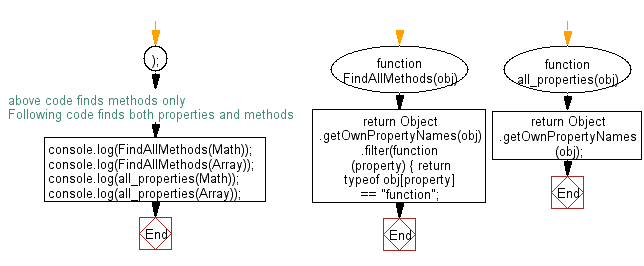# JavaScript: Print all the methods in an JavaScript object

## JavaScript Object: Exercise-11 with Solution

Write a JavaScript function to print all the methods in an JavaScript object.
Test Data:
console.log(all_properties(Array));
["length", "name", "arguments", "caller", "prototype", "isArray", "observe", "unobserve"]

Sample Solution: -

HTML Code:

``````<!DOCTYPE html>
<html>
<meta charset="utf-8">
<title>JavaScript function to display all methods in a object.</title>
<body>

</body>
</html>
```
```

JavaScript Code:

``````function FindAllMethods(obj) {
return Object.getOwnPropertyNames(obj).filter(function(property) {
return typeof obj[property] == "function";
});
}
//above code finds methods only
//Following code finds both properties and methods
console.log(FindAllMethods(Math));
console.log(FindAllMethods(Array));
function all_properties(obj)
{
return Object.getOwnPropertyNames(obj);
}

console.log(all_properties(Math));

console.log(all_properties(Array));
```
```

Sample Output:

```["abs", "acos", "acosh", "asin", "asinh", "atan", "atanh", "atan2", "ceil", "cbrt", "expm1", "clz32", "cos", "cosh", "exp", "floor", "fround", "hypot", "imul", "log", "log1p", "log2", "log10", "max", "min", "pow", "random", "round", "sign", "sin", "sinh", "sqrt", "tan", "tanh", "trunc"]
["isArray", "from", "of"]
["abs", "acos", "acosh", "asin", "asinh", "atan", "atanh", "atan2", "ceil", "cbrt", "expm1", "clz32", "cos", "cosh", "exp", "floor", "fround", "hypot", "imul", "log", "log1p", "log2", "log10", "max", "min", "pow", "random", "round", "sign", "sin", "sinh", "sqrt", "tan", "tanh", "trunc", "E", "LN10", "LN2", "LOG10E", "LOG2E", "PI", "SQRT1_2", "SQRT2"]
["length", "name", "prototype", "isArray", "from", "of"]
```

Flowchart:Live Demo:

Click on the Codepen logo at the top right corner of the code snippet below to view the output.

See the Pen javascript-object-exercise-11 by w3resource (@w3resource) on CodePen.

Improve this sample solution and post your code through Disqus

What is the difficulty level of this exercise?

﻿

## JavaScript: Tips of the Day

Returns the symmetric difference between two arrays, after applying the provided function to each array element of both

Example:

```const tips_symmetricDifference = (x, y, fn) => {
const sA = new Set(x.map(v => fn(v))),
sB = new Set(y.map(v => fn(v)));
return [...x.filter(x => !sB.has(fn(x))), ...y.filter(x => !sA.has(fn(x)))];
};

console.log(tips_symmetricDifference([3.5, 5.5], [5.5, 7.5], Math.floor));
```

Output:

```[3.5, 7.5]
```